# Exploratory Data Analysis### Exploratory Data Analysis (EDA)

EDA stands for Exploratory Data Analysis. It is a process of analyzing and summarizing a dataset in order to understand its characteristics and relationships between variables. EDA is typically the first step in the data science process, and it involves a lot of visualizing and summarizing the data using statistical techniques. The goal of EDA is to identify patterns and trends in the data that can help inform further analysis and modeling.

EDA typically involves the following steps:

1. Importing and cleaning the data
2. Visualizing the data
3. Summarizing the main characteristics of the data
4. Identifying relationships and patterns in the data

1. Importing and cleaning the data

Importing and cleaning the data is an essential step in the Exploratory Data Analysis (EDA) process. This step involves bringing the data into your analysis environment and preparing it for further analysis.
There are several key tasks involved in importing and cleaning the data:

• Importing the data: This involves reading the data into your analysis environment from a file or database. This can be done using a variety of tools, such as CSV readers, SQL queries, or spreadsheet software.
• Checking the data: After the data is imported, it is important to check that it has been read incorrectly and that there are no errors. This can involve checking the data types, the number of rows and columns, and the values in each column.
• Cleaning the data: Once the data is imported and checked, the next step is to clean it up. This can involve a variety of tasks, such as:
1. Removing duplicates: If there are duplicate rows in the data, they should be removed to avoid double-counting.
2. Handling missing values: If there are missing values in the data, you will need to decide how to handle them. This might involve imputing the missing values, or simply dropping rows with missing values.
3. Handling outliers: If there are extreme values in the data that are significantly outside the range of the rest of the data, they may need to be handled or removed.

By importing and cleaning the data properly, you can ensure that you have a high-quality dataset that is ready for further analysis.

2. Visualizing the data

Visualizing the data is an important step in the Exploratory Data Analysis (EDA) process, as it can help you gain insights into the characteristics and patterns of your data. Visualization involves using graphs, plots, and other types of visual representations to explore and understand the data.
There are many different types of visualizations that can be used to explore data, and the best choice will depend on the characteristics of your data and the questions you are trying to answer.
Some common types of visualizations include:

• Scatter plots: Scatter plots are used to visualize the relationship between two variables. They are particularly useful for identifying trends and patterns in the data.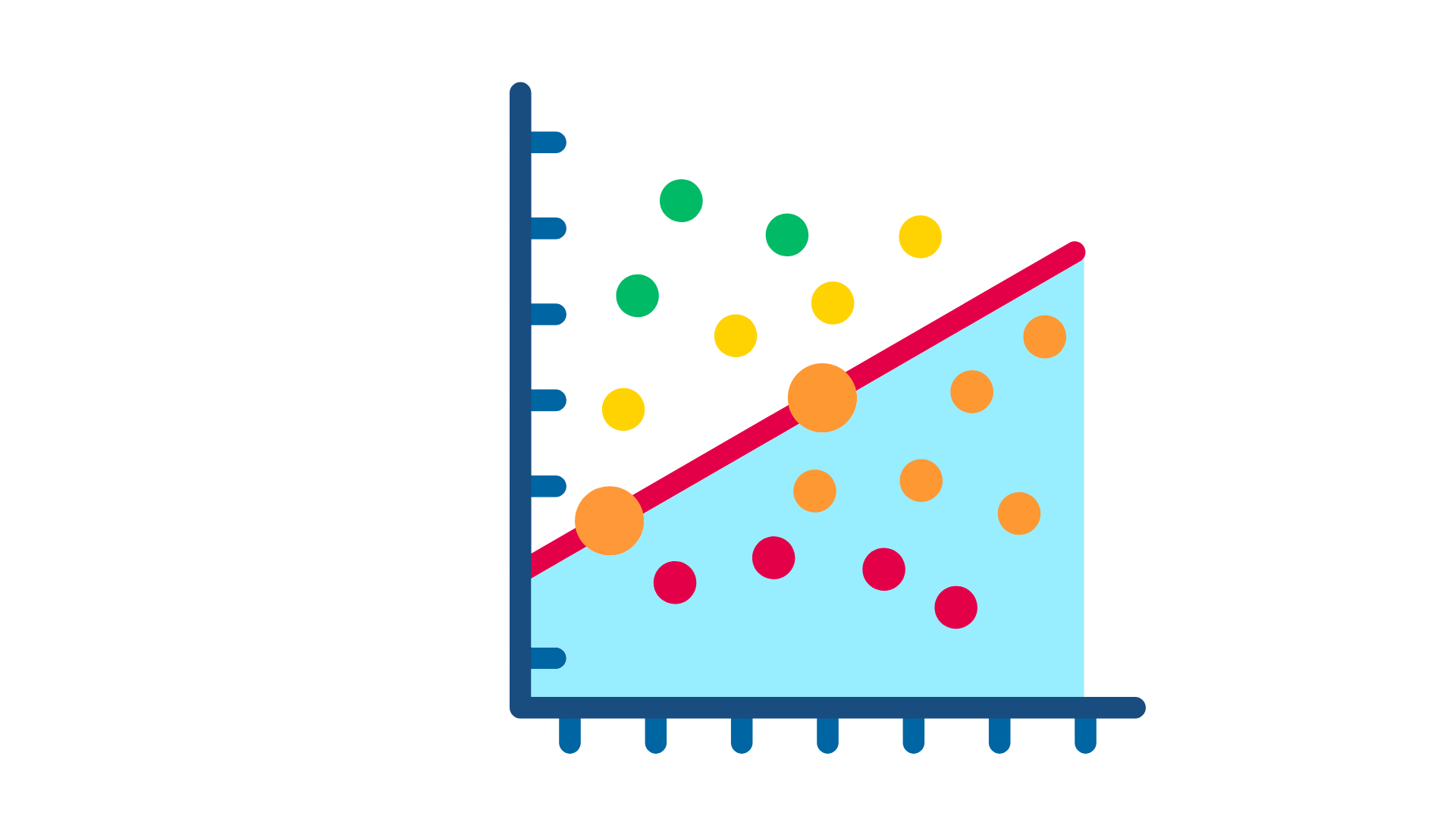• Line plots: Line plots are used to visualize trends over time. They can be used to show how a variable changes over a period of time, or how it compares to other variables.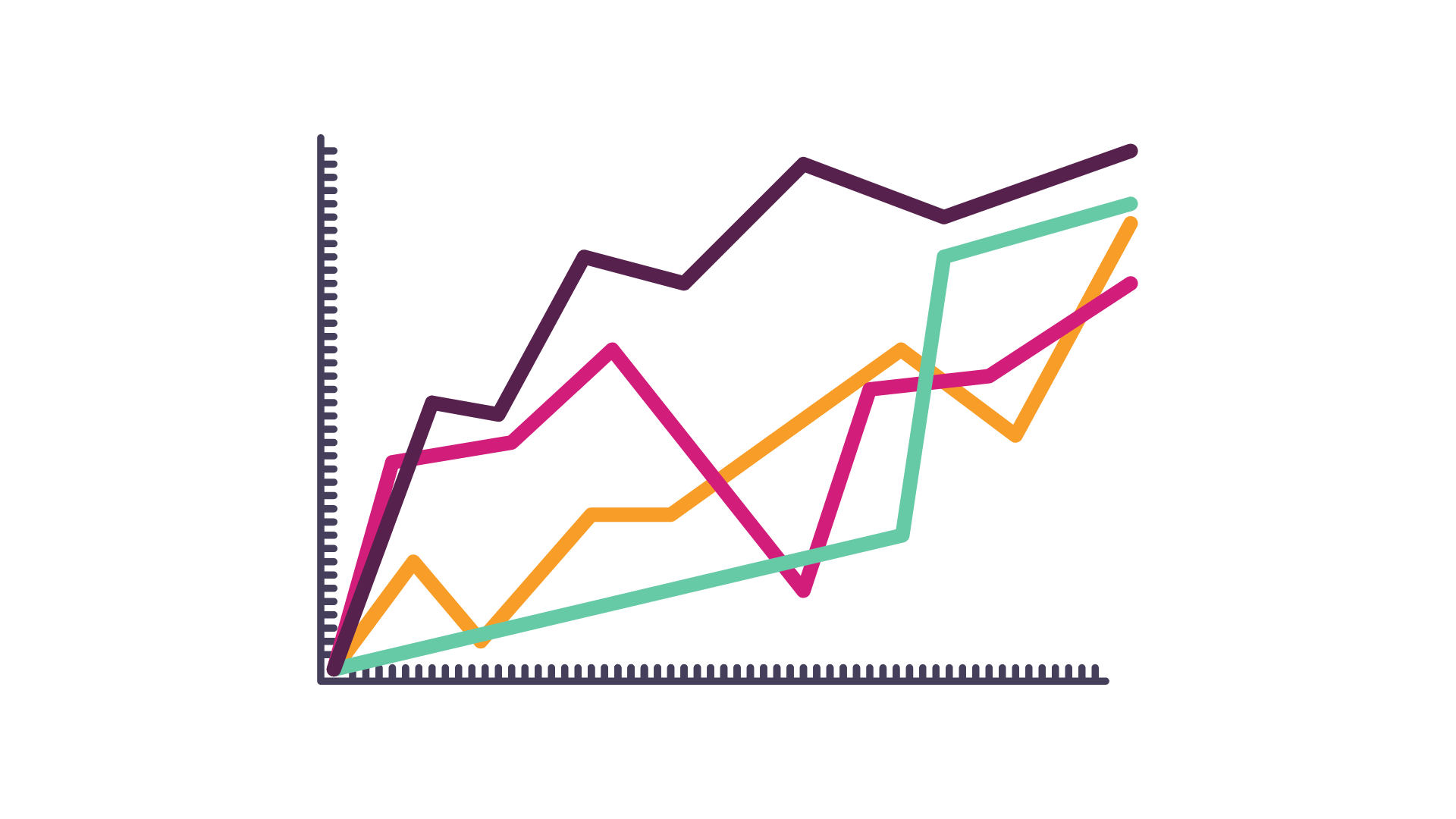• Histograms: Histograms are used to visualize the distribution of a single variable. They show the frequency of different values in the data and can help you identify patterns and outliers.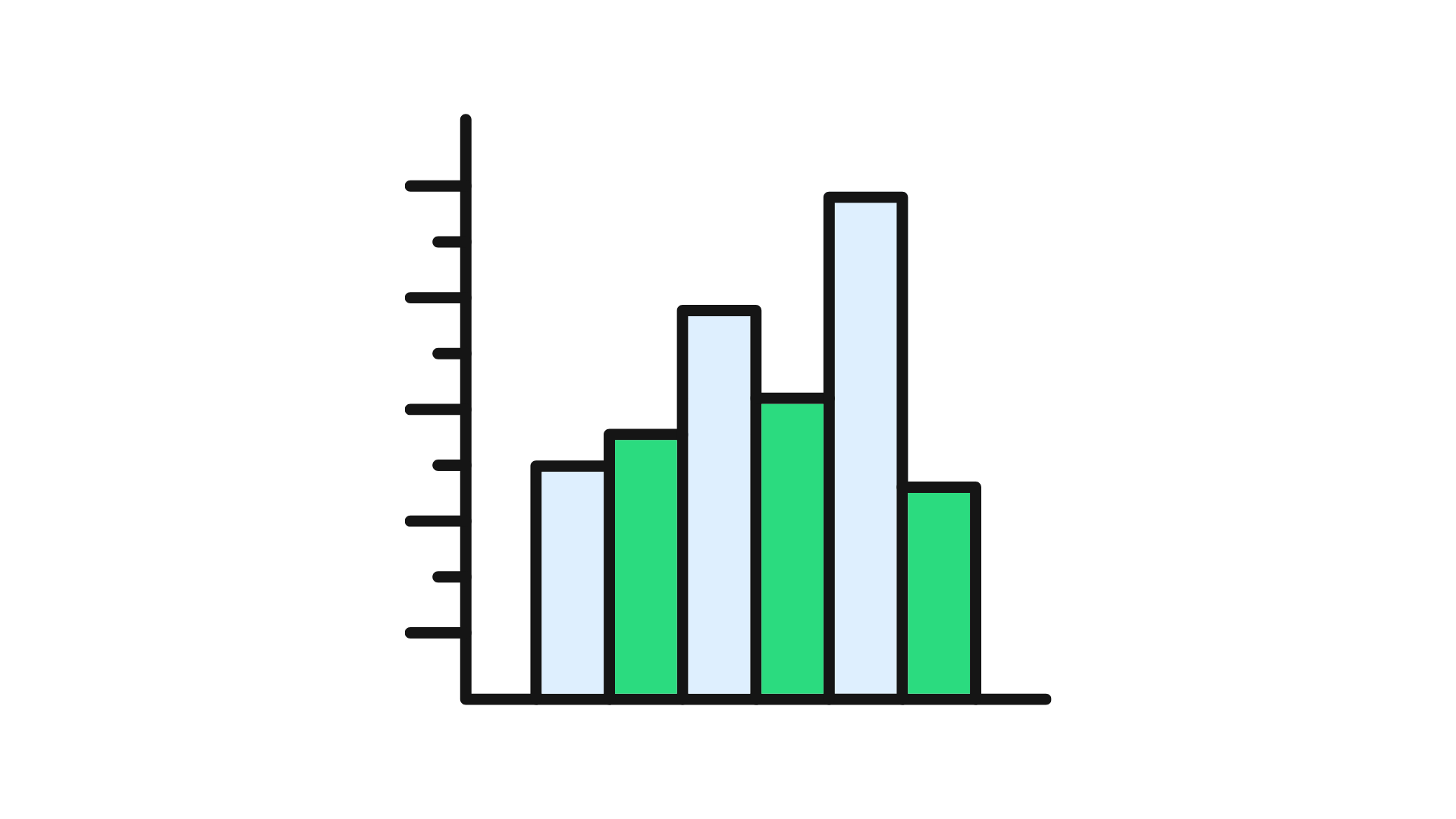Overall, visualization is a powerful tool for exploring and understanding data, and it can help you gain valuable insights and identify potential hypotheses or questions that can be further tested and explored.

3. Summarizing the main characteristics of the data

Summarizing the main characteristics of the data is an important step in the Exploratory Data Analysis (EDA) process, as it can help you better understand the overall structure and distribution of your data. There are many different ways to summarize the data, and the appropriate method will depend on the nature of the data and the questions you are trying to answer.
Some common techniques for summarizing the data include:

• Descriptive statistics: These statistics provide a summary of the main characteristics of the data, such as the mean, median, mode, and standard deviation.• Percentiles: Percentiles divide the data into 100 equal parts and can be used to identify the distribution of the data. For example, the 50th percentile is the median of the data.

• Box plots: Box plots provide a summary of the distribution of a continuous variable, and can be used to identify the minimum, first quartile, median, third quartile, and maximum values of the data.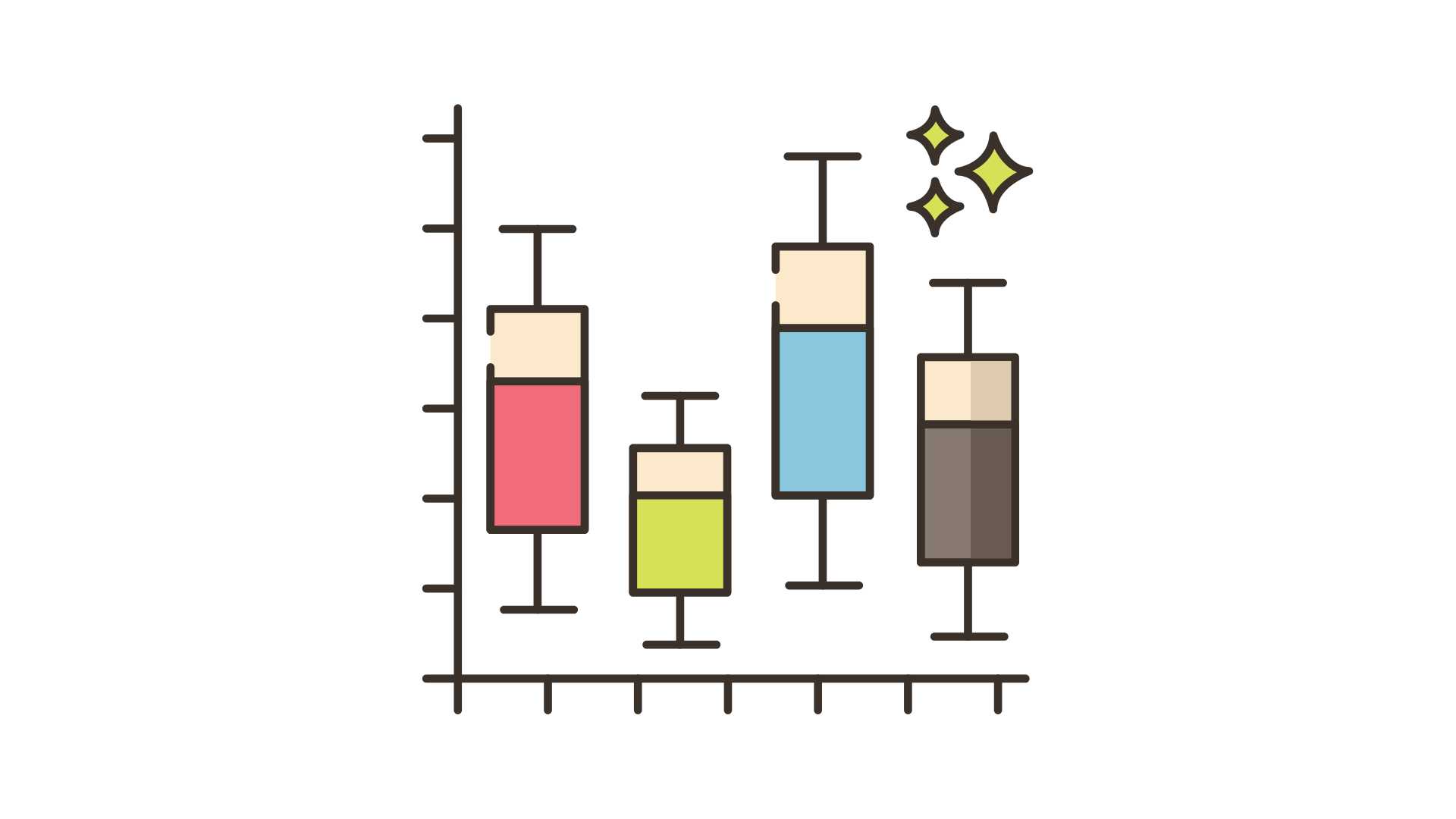By summarizing the main characteristics of the data, you can gain a better understanding of the overall structure and distribution of the data, and identify patterns and trends that may not be immediately apparent from the raw data. This can inform your next steps and help you better understand your data.

4. Identifying relationships and patterns in the data

Identifying relationships and patterns in the data is an important step in the Exploratory Data Analysis (EDA) process, as it can help you better understand the underlying structures and patterns in the data. There are many different techniques that can be used to identify relationships and patterns in the data, and the appropriate method will depend on the nature of the data and the questions you are trying to answer.
Some common techniques for identifying relationships and patterns in the data include:

• Scatter plots: These plots show the relationship between two variables and can be used to identify correlations and trends.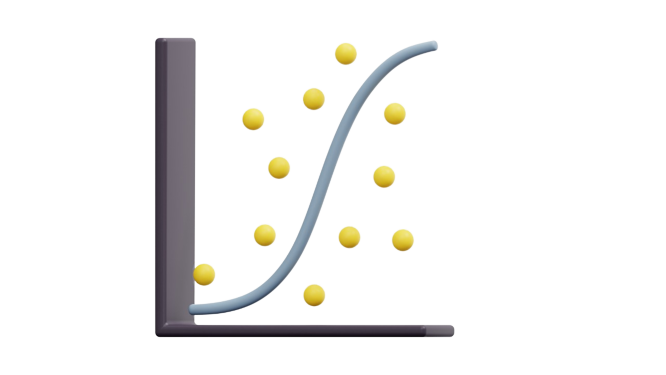• Correlation analyses: These analyses measure the strength and direction of the relationship between two variables. For example, a strong positive correlation indicates that as one variable increases, the other variable also increases.
• Regression analyses: These analyses examine the relationship between a dependent variable and one or more independent variables. They can be used to identify the strength and direction of the relationship, and to predict the value of the dependent variable based on the values of the independent variables.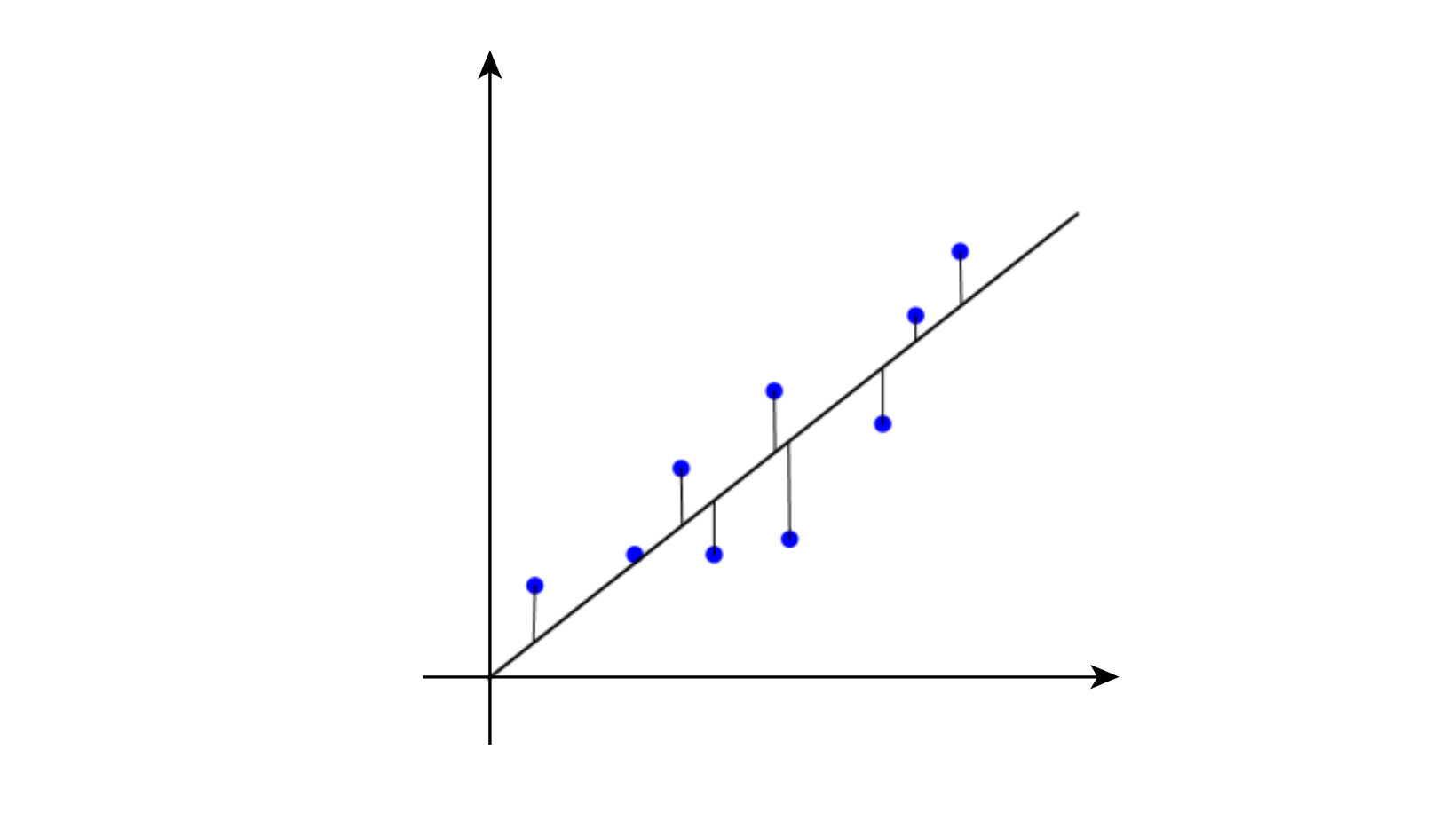By identifying relationships and patterns in the data, you can gain a better understanding of the underlying structures and patterns in the data, and identify potential hypotheses or questions that can be further tested and explored. This can inform your next steps and help you better understand your data.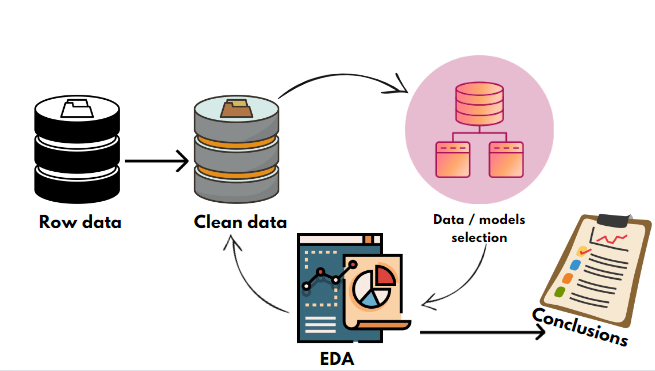One of the key goals of EDA is to better understand the characteristics of your data. You can use visualization tools, such as graphs and plots, to explore the data and identify patterns and relationships. Some common types of plots include scatter plots, line plots, and histograms.

You can also use descriptive statistics, such as the mean, median, and standard deviation, to summarize the main characteristics of the data. By examining the distribution of different variables and identifying relationships and patterns, you can gain valuable insights into your data and inform your next steps.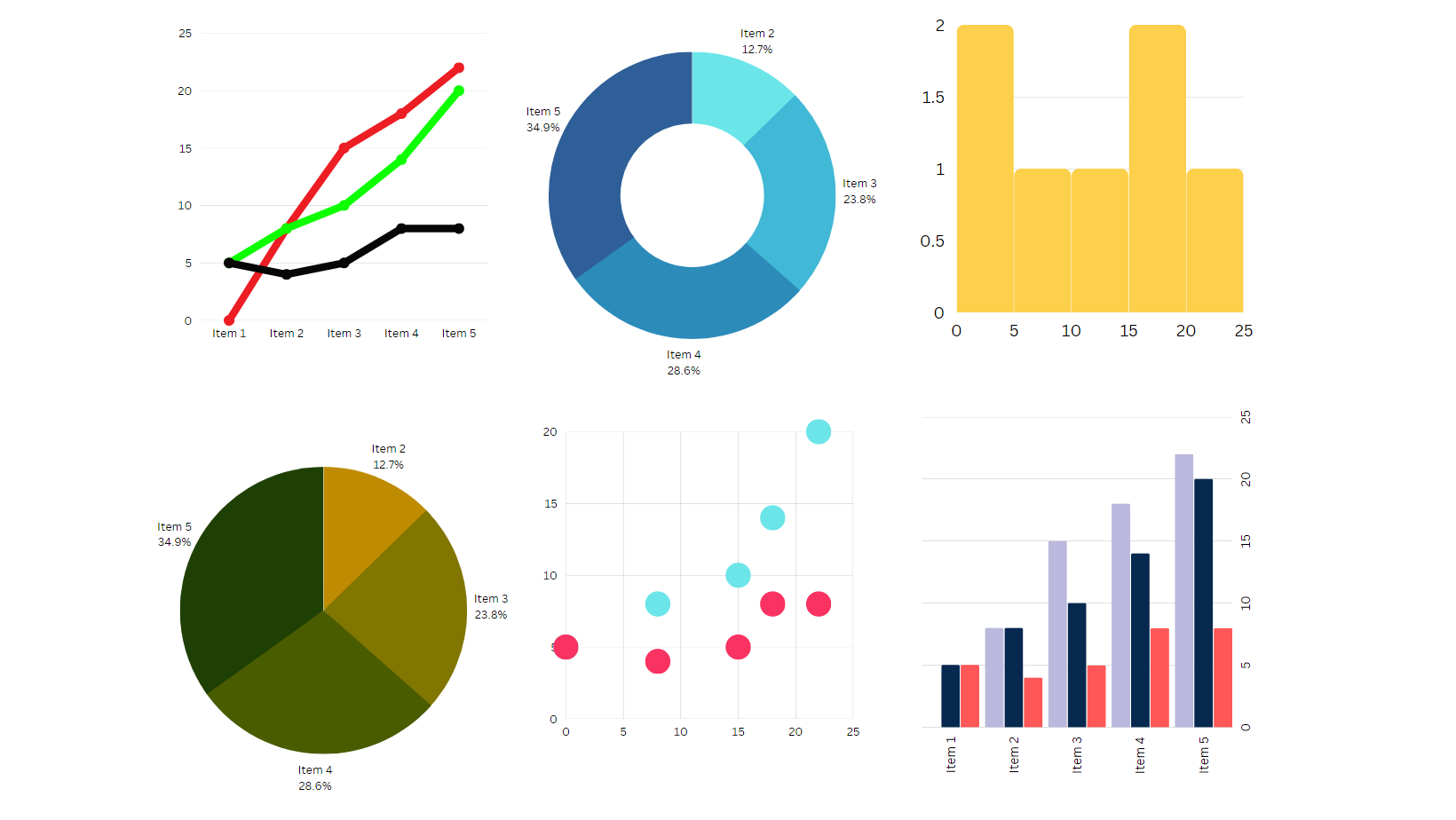Overall, the goal of EDA is to gain a better understanding of the data and to identify potential hypotheses or questions that can be further tested and explored. By using visualization and statistical analysis, you can better position yourself to make informed decisions and draw accurate conclusions about your data.